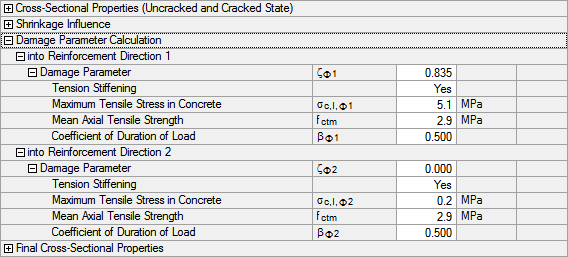# RF-CONCRETE Surfaces – Online Manual Version 5

Online manuals, introductory examples, tutorials, and other documentation.

# 2.7.10.7 Calculation of distribution coefficient

#### Calculation of distribution coefficient

The maximum stress in the uncracked state is:

We assume a long-term loading:

Taking Tension Stiffening into account, the distribution coefficient is calculated according to the following equation:

• for σmax,φ1 > fctm:

• for σmax,φ1 ≤ fctm:

In the example, the maximum tension stress in the concrete is larger than the concrete tensile strength.

Thus, the distribution coefficient is:Figure 2.123 Calculation of distribution coefficient (damage parameter)